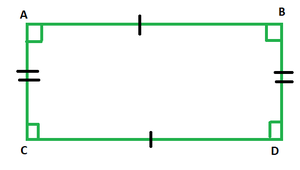GeeksforGeeks App
Open AppBrowser
Continue

# If the length of a rectangle is increased by 50%, by what percentage would be the width have to be decreased to maintain the same area?

Except for liquids, everything in our environment has a defined form. Everything has a form, from our phones and computers to our cabinets, water bottles, microwave ovens, kitchen bowls, water tanks, LPG pipes, and so on. Obviously, some of these items are utilized to store our belongings. In Mathematics, such forms are classified according to particular characteristics and researched in order to either build additional shapes similar to them or improve current ones by covering or adorning them. In addition, their storage capacity is studied in mathematics in order to use them to hold things.

### Mensuration

Mensuration is the field of mathematics concerned with the calculation of geometrical dimensions and dimensional values. It is the act of measuring anything, whether it be the length, width, height, area, or any other characteristic relevant to all geometrical objects we see and utilize on a daily basis.

### Rectangle

A rectangle is a quadrilateral with equal parallel sides and all angles measuring 90 degrees. It’s a two-dimensional form with only two measurements: length and width. The rectangle ABCD is depicted in the diagram below, with 90-degree angles on each side and equal-length parallel sides.The sides AB and CD denote the rectangle’s length, while AC and BD represent its width. The sum of the internal angles of rectangle ABCD is 360 degrees, just like any other quadrilateral.

### Area of a Rectangle

The area of a rectangle is calculated by multiplying both of its dimensions, which must be of the same unit. If the area of rectangle ABCD in the illustration above were computed, it would come out to (AB × CD) sq. units.

Area of a rectangle = l × b square units.

### If the length of a rectangle is increased by 50%, by what percentage would be the width have to be decreased to maintain the same area?

Solution:

We know that the area of a rectangle is equal to l × b sq. units.

New length = l + 50% of l = 3l/2

⇒ New area = 3lb/2

Let’s call the new width  b0.

⇒  l × b = 3l/2 × b0

⇒ b0 = 2b/3

⇒ Percentage decrease in width == 100/3

= 33.33%

As a result, the width must be reduced by 33.33 percent.

### Similar Problems

Question 1. The length of a rectangle is increased by 80%. By what percentage would be the width have to be decreased to maintain the same area?

Solution:

We know, area of a rectangle = l × b sq. units.

New length = l + 80% of l = 9l/5

⇒ Area of rectangle with new length = 9lb/ 5

Let the new breadth be b0.

⇒  l × b = 9l/5 × b0

⇒ b0 = 5b/9

⇒ Percentage decrease in width == 400/9

= 44.5%

Hence the breadth has to be decreased by 44.5%.

Question 2. The length of a rectangle is increased by 60%. By what percentage would be the width have to be decreased to maintain the same area?

Solution:

We know, area of a rectangle = l × b sq. units.

New length = l + 60% of l = 8l/5

⇒ Area of rectangle with new length = 8lb/ 5

Let the new breadth be b0.

⇒  l × b = 8l/5 × b0

⇒ b0 = 5b/8

⇒ Percentage decrease in width == 300/8

= 37.5%

Hence the breadth has to be decreased by 37.5%.

Question 3. The length of a rectangle is decreased by 70%. By what percentage would be the width have to be increased to maintain the same area?

Solution:

We know, area of a rectangle = l × b sq. units

New length = l – 70% of l = 3l/10

⇒ Area of rectangle with new length = 3lb/ 10

Let the new breadth be b0.

⇒  l × b = 3l/10 × b0

⇒ b0 = 10b/3

⇒ Percentage increase in width == 700/3

= 233.4%

Hence the breadth has to be decreased by 233.4%.

Question 4. The length of a rectangle is increased by 20%. By what percentage would be the width have to be decreased to maintain the same area?

Solution:

We know, area of a rectangle = l × b sq. units.

New length = l + 20% of l = 6l/5

⇒ Area of rectangle with new length = 6lb/ 5

Let the new breadth be b0.

⇒  l × b = 6l/5 × b0

⇒ b0 = 5b/6

⇒ Percentage decrease in width == 100/6

= 16.67%

Hence the breadth has to be decreased by 16.67%.

Question 5. The length of a rectangle is increased by 40%. By what percentage would be the width have to be decreased to maintain the same area?

Solution:

We know, area of a rectangle = l × b sq. units.

New length = l + 40% of l = 7l/5

⇒ Area of rectangle with new length = 7lb/ 5

Let the new breadth be b0.

⇒  l × b = 7l/5 × b0

⇒ b0 = 5b/7

⇒ Percentage decrease in width == 200/7

= 28.57%

Hence the breadth has to be decreased by 28.57%.

My Personal Notes arrow_drop_up
Related Tutorials